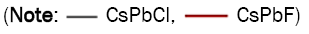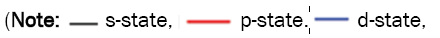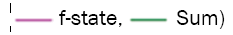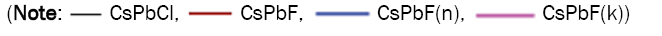All submissions of the EM system will be redirected to Online Manuscript Submission System. Authors are requested to submit articles directly to Online Manuscript Submission System of respective journal.

# Structural, Electronic, Mechanical, and Optical Properties of CsPbX3 (X=Cl, F) for Energy Storage and Hybrid Solar Cell Applications

Jalil Ur Rehman*, Huma Khalid, Muhammad Usman, M. Bilal Tahir, Abid Hussain

Department of Physics, Khwaja Fareed University of Engineering and Information Technology, Rahim Yar Khan, Pakistan

*Corresponding Author:
Jalil Ur Rehman
Department of Physics,
Khwaja Fareed University of Engineering and Information Technology,
Rahim Yar Khan,
Pakistan
E-mail:
jaliliub@gmail.com

Received: 25-Jul-2022, Manuscript No. JOMS-22-70152; Editor assigned: 29-Jul-2022, PreQC No. JOMS-22-70152 (PQ); Reviewed: 12-Aug-2022, QC No. JOMS-22-70152; Revised: 19-Aug-2022, Manuscript No. JOMS-22-70152 (R); Published: 26-Aug-2022, DOI: 10.4172/2321-6212.10.8.001.

Visit for more related articles at Research & Reviews: Journal of Material Sciences

## Abstract

In this paper, two perovskite crystals, CsPbX3(X=Cl, F), have investigated structural, optoelectronic, and elastic properties in detail using density functional theory-based CASTEP code. Estimated lattice parameters match very well with previous theoretical and experimental results. These materials have semiconducting properties, according to the estimated electronic structure profile. Pugh's criterion predicts the ductile/brittle of CsPbX3 (X=Cl, F), and our estimated elastic constants satisfy the structural stability criterion. The dielectric functions demonstrate that real and imaginary parts of compounds have similar abilities to retain energy. Such characteristics make them ideal for solar cells and energy storage systems. At high photon energies, these materials also act as super luminous materials.

#### Keywords

Perovskite crystals; Photovoltaic devices; Density functional theory; Halogens

#### Introdcuction

Solar energy is among most desirable renewable energy sources on earth. Simple words, it is an alternate energy source that can solve current energy issues and implement various energy sources . Solar cells, which convert sunlight directly into electricity with little byproducts or greenhouse gas emissions, are one potential source of this type of renewable energy . Halide perovskites have gained a lot of attention in current history for developing electronic devices like solar cells and light-emitting diodes. Several projects have already been launched to find lead-free perovskite absorber compounds. Many methods have been used to enhance their energy conversion efficiency, including improving the effectiveness and regularity of perovskite films and bandgap tuning. Perovskite is recently attracted a lot of attention in the solar cell field. The ABX3 is the formula of Hybrid Organic-Inorganic Perovskites (HOIPs), in which A-site is filled with small organic ions, the B-site with divalent metallic atom, and the X-site are held with halogens [3,4]. They also have outstanding semiconducting and light absorption capabilities, making them attractive candidates in optoelectronic device applications, particularly in photovoltaic systems, where they have a power conversion efficiency of more than 20% [5,6]. As a result, different forms of photovoltaic devices, like quantum dots and perovskite solar cells, are linked to solar cells in modern technologies. Perovskite solar cells, often known as "third generation solar cells," have developed as a feasible, environmentally sustainable, and renewable alternative to photovoltaics with the potential to solve critical issues in the areas of global energy . Furthermore, most current perovskite which are based on solar cells have a conversion efficiency of up to 25.5 per cent. Focusing on a light lens in a small photocell recently highlighted the theoretical performance of more than 46% solar inverter based on halide perovskites. Solar cells' conversion efficiency is determined by optical characteristics of materials or their ability to absorb light, which is determined by materials' band gap. Band gap of solar cell materials affects their efficiency, whereas physical characteristics and phase transition influence band gap. Most of the materials in this class that have outstanding achievements contain lead (Pb). As a result, the toxicity of Pb is a priority for using these materials in practice . Lead-based metal halide perovskites break down in ambient situations, which is environmentally harmful [9,10]. At different temperatures, these compounds demonstrate flexibility in changing crystalline forms. The temperature ranges for phase transition in CsPbX3 (Cl, F) are determined by the halogen atom linked . In this study, we focus on two perovskites, CsPbX3 (X=Cl, F), and use Density Functional Theory (DFT) to compare and contrast their properties, giving special attention to the impact of the third atom shift. The work is organized in the following way: first, we explain the calculations; second, we provide the structural properties of the analyzed materials; third, we describe and discuss the obtained results; and finally, we end with a summary.

#### Materials and Methods

Nickel electroforming

In this research, all computations are carried out using the full potential linearized augmented plane wave approach (DFT) in a density functional theory framework. CASTEP is used to perform all of the computations, which are run within Material Studio [12,13]. The 2 × 2 × 2 unit cells containing atoms are created to produce the desired pure cubic CsPbX3(X=Cl, F) metal halides. The electronic configurations of atoms in compound is: Cs:1s2, 2s2, 2p6, 3s2, 3p6, 3d10, 4s2, 4p6, 4d10, 5s2, 5p6, 6s1, Cl: 1s2, 2s2, 2p6, 3s2, 3p6, F: 1s2, 2s2, 2p5, Pb: 1s2 2s2 2p6 3s2 3p6 3d10 4s2 4p6, 4d10 4f14, 5s2, 5p6, 5d10,6s1. Both materials, CsPbX3(X=Cl, F), belong to the Pm3m space group. In the pure sample, 4 × 4 × 4 k-points were used to ensure convergence criterion for calculation and geometry optimization. For CsPbX3 (X=Cl, F), the plane wave cut-off energy of the wave function is expanding at 244.9 eV. The residual forces act during geometry optimization at atoms; it is 0.3 eV/A0. The maximum strain amplitude was set at 0. 003. Based on DFT Kohn–Sham orbitals, the calculation of optical properties is performed by the CASTEP software. CsPbX3 (X=Cl, F) compound atoms are situated as the Wyckoff coordinates 1a (0,0,0), 1b (0.5,0.5,0.5), 3c (0.5,0.5,0), respectively. For compound, total energy per atom of CsPbX3 (X=Cl, F) is 2.0 × 10-5 eV/atom, maximum force is 0.05 eV/Å. The maximum stress of the compound was taken at 0.1 GPa, and the maximum displacement was at 0.002 Å. Putting the total energy vs. volume, Murnaghan's state equation provides the compound's lattice constants and bulk modulus.

#### Results and Discussion

Structural analysis

The geometry optimization of both unit cells CsPbX3 (X=Cl, F) provides calculations like volume, lattice constant, and bulk modulus. Because these perovskites are sensitive to temperature, CsPbX3 (X=Cl, F) shows up in different stages at different temperatures. As shown in Figures 1a and 1b, structural explanation of CsPbX3 (X=Cl, F) is cubic with Pb in the middle, six halogens at faces, and Cs in the cubic corner. The orthorhombic phase of this compound is stable at room temperature . The structure distorts whenever the temperature is raised, and the material enters the tetragonal phase.

Optimized lattice parameter values of previous and current studies of the compounds are given in Table 1.

Lattice parameters (Å) Volume (Å 3)
a b c
Previous study CsPbCl3  5.732 5.732 5.732 188.32
CsPbF3 4.774 4.774 4.774 108.8
Current study CsPbCl3 5.818 5.818 5.818 196.93
CsPbF3 5.054 5.054 5.054 129.09

Table 1. Lattice parameter and volume of CsPbX3 (X=Cl, F).

The previously reported value of the CsPbCl3 lattice parameter was a=b=c=5.732, closer to the current value, with only a 0.08 difference. The previously reported value of the CsPbF3 lattice parameter was a=b=c=4.77A, which is quite near to the present study, and the only difference between these values is 0.28.

Electronic properties

We move on to electronic properties of these two crystals after confirming reasonability of optimized crystal structures. Firstly, we started with a bandgap and then calculated TDOS and PDOS. The bandgap structure indicates valence electron contributions of respective materials.

In contrast, PDOS allows for the intra-band and inter-band transitions between sub-states like s, p, d, and f-states of a material. The valence band and conduction band are two different types of energy bands. Valence band lies below Fermi level (EF), while the conduction band is above it. The band gap will be direct if the VBM happens precisely upon CBM.

In another instance, an indirect band gap forms when the CBM and VBM are not perfectly aligned. Band structures as calculated can be seen in Figures 2a and 2b. CsPbX3 (X=Cl, F) has a direct band gap between its conduction and valence bands at R, where the conduction band gap is minimum and valence band gap is highest. Current calculated band gap of CsPbCl3 and CsPbF is 2.370 eV and 3.028 eV, and the previously reported value of the band gap is 2.168 eV and 5.344 eV. The band gap of CsPbF3 is slightly more significant than CsPbCl3. The horizontal dashed line in the band structure represents Fermi level EF.

The density of state theory explains the nature of the band gap energy of CsPbX3(Cl, F) compounds. In Figure 3, the total density of the state is displayed, which explains the nature of the compounds. TDOS plots for these compounds reveal that these materials have semiconducting characteristics. The TDOS sharp peak of CsPbCl3 shows at -15.67 eV, and the minimum peak shows at 11.68 eV. The TDOS sharp peak of CsPbF3 shows at -2.15 eV, and the minimum peak shows at 9.68 eV. PDOS graphs show how atoms and their various states contribute to the band gap energy of CsPbX3 (Cl, F) compounds.

Figure 3: The total density of states of CsPbCl3 and CsPbF3.So range of energy in TDOS and PDOS plots is -10 eV to 20 eV. In Figure 4a, shows PDOS graph of CsPbCl3 (Figure 4a) and CsPbF3 (Figure 4b). In PDOS graphs, the main peak of s-state in CsPbCl3 and CsPbF3 are occurs at 6.30 eV and 7.32 eV, for p-state are at -6.98 eV and -2.08 eV. The maximum peaks of CsPbX3(Cl, F) compounds are at 19.08 and 20.02.

Figure 4: Partial density of states of (a) CsPbCl3 and (b) CsPbF3.Elastic properties

Despite the cubic symmetry of the compounds under study, we will calculate only three elastic parameters, C11, C12, and C44, to describe their mechanical properties . Mechanical characteristics of CsPbX3(Cl, F) compounds were investigated using the CASTEP code. According to studies, elastic constants are stable and meet mechanical stability criteria for cubic crystals. All of the compositions clearly meet well-known Born stability criteria, which are as follows: C11−C12>0, C11>0, C44>0, C11+2C12>0 . With the help of computed elastic constants, most significant mechanical characteristics of a compound are given in Table 2. Using the following relations, we can find the terms mentioned below:

B=(C11+2C12)/3-------- (1)

A=(2C4)/(C11–C12) --------- (2)

E=(9BG)/(3B+G) --------- (3)

v=(3B-2G)/2(2B+G) ---------------- (4)

G=(Gv+GR)/2 ---------------- (5)

Gv=(C11–C12+3C44)/5 -------------------(6)

GR=5C44(C11–C12)/4C44+3(C11–C12) -----------------(7)

The bulk modulus is one of its most critical mechanical parameters that indicate a material's stiffness. Another essential parameter for defining the bonding nature as well as the plasticity of material is Poisson's ratio. The material ductility or brittleness can be determined using the B/G balance. Material is brittle if ratio is less than 1.75 and ductile if larger than 1.75. According to Pugh's criteria, CsPbCl3 and CsPbF3 are brittle. If Poisson's ratio is more than 0.26, material is brittle, but if it is less, the compound is ductile. So both compounds are breakable. The anisotropic factor is determined for both compounds CsPbCl3 and CsPbF3; these values are mentioned in Table 2. If factor A equals one, the material is isotropic; otherwise, it is anisotropic . The value of A for CsPbCl3 and CsPbF3 are not equal to one, so compounds are anisotropic. First-principles computations calculate single crystals for both compounds' elastic constants C11, C12, and C44.

 Parameters CsPbCl3 CsPbF3 C11 37.5249 32.1996 C12 6.0578 -19.434 C44 7.23385 13.441 A 0.76171 0.52965 G 10.6337 18.3913 E 26.2731 -31.3841 V 0.23537 -1.85323 B/G 1.556 0.1208

Table 2. Elastic constants, bulk modulus, shear modulus and Young’s modulus, as well as the B/G ratio and anisotropy ratio of CsPbX3 (Cl, F).

Optical properties

Among all photovoltaic materials, CsPbX3 (Cl, F) compounds are the best light absorbers [19,20]. Therefore, it is in the interest of all scientists to increase their understanding of these materials so that longer-lasting and more productive solar cells and other solar systems can be developed [21,22]. To understand how these CsPbX3 (Cl, F) compounds respond to incident photons, we may calculate different optical parameters. Using dielectric functions, which are given by ε1(u) and ε2(u), respectively, we can explain the optical properties of these cubic compounds shown in Figures 5a-5f. The following relations are used to calculate various optical properties:

ε(ω)=ε1(ω)+iε2(ω)-------------(a)

n(ω)=[ε1(ω)/2+{ε21(ω)+ε21(ω)}1/2/2]1/2 … … ……. (b)

L(ω)=-Im(ε(ω)−1)=ε2(ω) /ε1(ω)2+ε2(ω)2 … … … … … (c)

I(ω)=21/2ω[{ε12(ω)+ε12(ω)}1/2-ε1(ω)]1/2 … … … … …. (d)

R(ω)=(n+ k–1)/((n+i k+1) … … … …(e)

K(ω)=I(ω)/2 ω … …. (f)

In Figure 5a, for CsPbCl3 the maximum peak value of reflectivity is 0.472 at 16.58eV and for CsPbF3 the highest value is 0.387 at 16.24 eV. With regards to photovoltaics, it is a fundamental optical property of materials. Figure 5b shows the highest peak value of absorption is 432581.96 occurs at 33.51 eV for CsPbCl3 and the sharp peak value of CsPbF3 is 324180.32 at 15.60 eV. Figure 5c represents refractive index of both compounds for CsPbCl3 highest peak value of the real part is 2.347, shown at 3.786 eV, and the sharp peak value for imaginary part is 1.362 at 14.46 eV. For CsPbF3, the highest peak value of the refractive index real part is 2.045 at 4.49 eV, and maximum peak value for imaginary part is 1.295 at 15.49 eV. In general, Covalent bonds have a greater refractive index as compared to ionic bonding . Figure 5d shows strong peak values of the CsPbCl3 real part, and imaginary part of dielectric function is 5.30 at 3.56 eV and 4.190 at 4.84 eV, respectively. For CsPbF3, the highest peak values of the real and imaginary parts are 4.028 at 4.2 eV and 3.140 at 14.89 eV, respectively. Optical conductivity is a characteristic of electron conduction caused by an applied electromagnetic field [24,25]. Figure 5e shows the conductivity; the CsPbCl3 maximum peak value of real and imaginary parts is 5.335 and 3.945. The sharp peak of the real part of CsPbCl3 shows at 14.01 eV and the imaginary part at 14.78 eV. The CsPbF3 maximum peak value of the real and imaginary part of conductivity is 5.707 at 14.97 eV and 3.827 at 15.86 eV, respectively. The energy loss function for CsPbX3 (Cl, F) compounds describes how energy is lost when incident photon's energy exceeds material's bandgap [26,27]. Figure 5f shows the loss function of both compounds, the sharp peak values of CsPbCl3 and CsPbF3 are 4.584 at 17.8 eV and 2.081 at 20.06 eV.

Figure 5: Optical properties of CsPbCl3 and CsPbF3 (a) Reflection (b) Absorption (c) Refractive index (d) Dielectric function (e) Conductivity (f) Loss function.#### Conclusion

The structural, electrical, optical, and elastic characteristics of cubic cesium halide perovskites CsPbX3(Cl, F) were calculated using the CASTEP code's first-principles calculations. These cesium halide perovskites are mechanically stable enough to classify as cubic crystals, and they exhibit semiconductor behavior due to their direct band gap. Both compounds represent anisotropic behavior according to anisotropic factors. In energy range of 0–40 eV, optical properties were examined. CsPbX3(Cl, F) have excellent properties, making them ideal for energy storage devices and solar cells. We hope our work opens the path for better performance for future experimental and theoretical research and access to various discoveries and innovations in materials science.